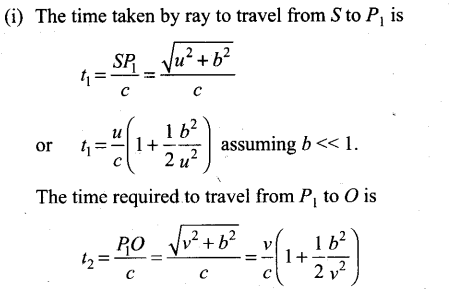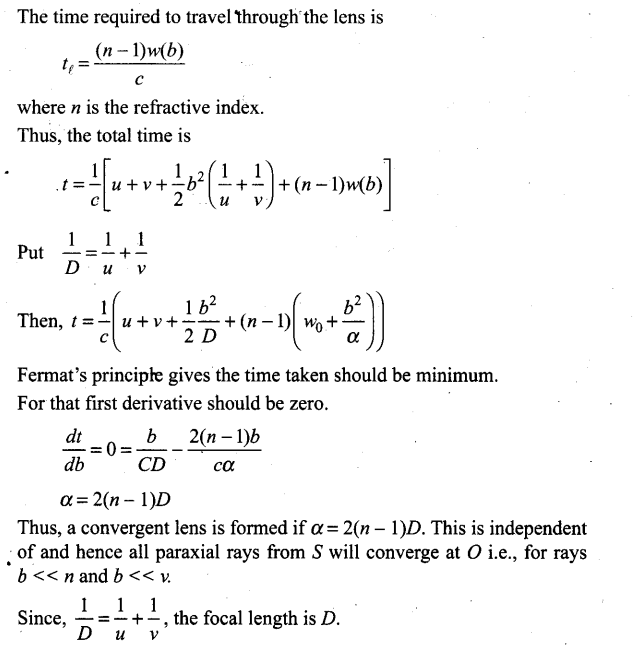# Ray Optics and Optical Instruments NCERT Exemplar Problems Solutions Physics

0
2402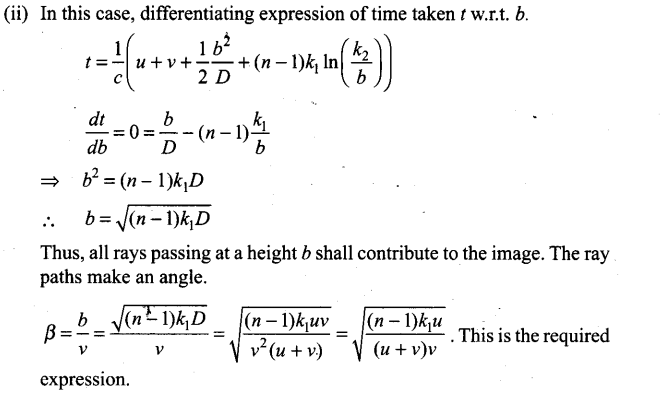## NCERT Exemplar Problems Class 12 Physics Chapter 9 Ray Optics and Optical Instruments

Multiple Choice Questions (MCQs)

Question 1. A ray of light incident at an angle d on a refracting face of a prism emerges from the other face normally. If the angle of the prism is 5° and the prism is mad? of a material of refractive index 1.5, the angle of incidence is
(a) 7.5° (b) 5° (c) 15° (d) 2.5°
Solution: (a)
Key concept:
In thin prisms, the distance between the refracting surfaces is ineligible and the angle of prism (A) is very small. Since A = r1 + r2, therefore if A is small then both r1 , and r2 are also small, and the same is true for i1 and i2.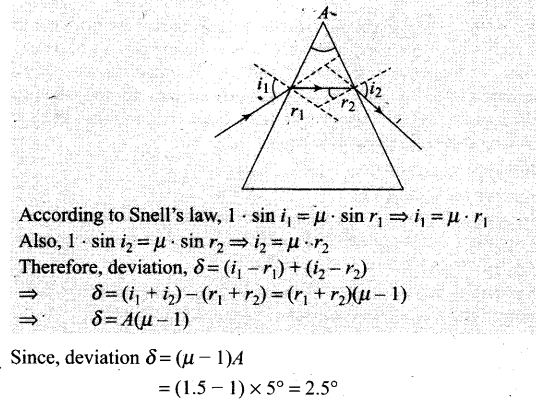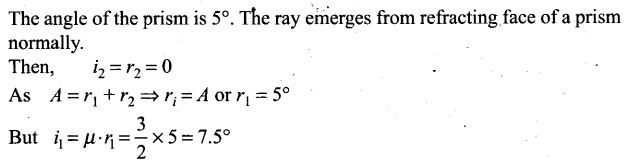Question 2. A‘ short pulse of white light is incident from air to a glass slab at normal incidence. After travelling through the slab, the first colour to emerge is
(a) blue (b) green (c) violet (d) red
Solution: (d) As velocity of wave is given by the relation v = f λ . When light ray goes from one medium to other medium, the frequency of light remains unchanged. Hence v ∝ λ or greater the wavelength, greater the speed.
The light of red colour is of highest wavelength and therefore of highest speed. Therefore, after travelling through the slab, the red colour emerges first.

Question 3. An object approaches a convergent lens from the left of the lens with a uniform speed 5 m/s and stops at the focus. The image
(a) moves away from the lens with an uniform speed 5 m/s
(b) moves away from the lens with an uniform acceleration
(c) moves away from the lens with a non-uniform acceleration
(d) moves towards the lens with a non-uniform acceleration
Solution: (c)
In our problem the object approaches a convergent lens from the left of the lens with a uniform speed of 5 m/s, hence the image will move away from the lens with a non-uniform acceleration, the image moves slower in the beginning and faster later on will move from F to 2F and when the object moves from 2F to F,the image will move from 2F to infinity. At 2F, the speed of the object and image will be equal.

Question 4. A passenger in an aeroplane shall
(a) never see a rainbow
(b) may see a primary and a secondary rainbow as concentric circles
(c) may see a primary and a secondary rainbow as concentric arcs
(d) shall never see a secondary rainbow
Solution: (b) As aeroplane is at higher altitude, the passenger in an aeroplane may see a primary and a secondary rainbow like concentric circles.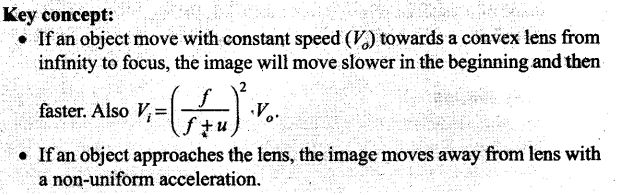Question 5. You are given four sources of light each one providing a light of a single colour—red, blue, green and yellow. Suppose the angle of refraction for a beam of yellow light corresponding to a particular angle of incidence at the interface of two media is 90°. Which of the following statements is correct if the source of yellow light is replaced with that of other lights without changing the angle of incidence?
(a) The beam of red light would undergo total internal reflection.
(b) The beam of red light would bend towards the normal while it gets refracted through the second medium.
(c) The beam of blue light would undergo total internal reflection.
(d) The beam of green light would bend away from the normal as it gets refracted through the second medium.
Solution: (c)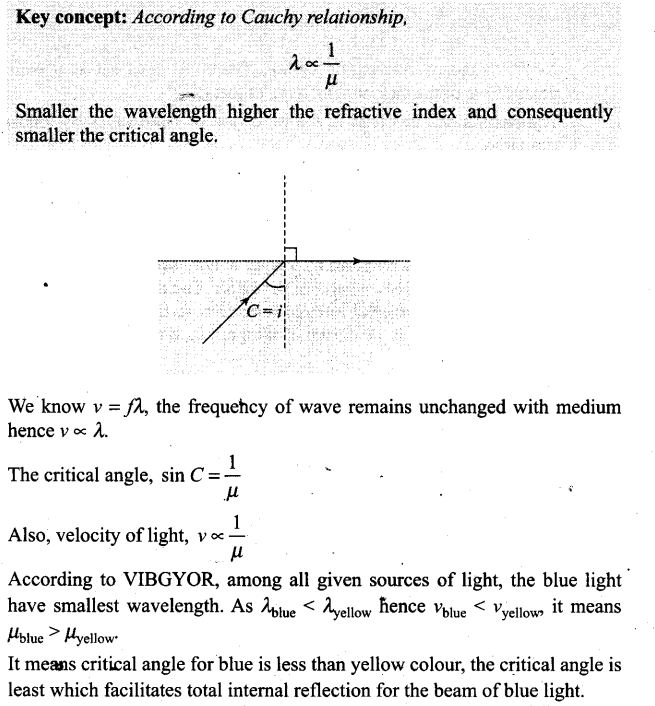Question 6. The radius of curvature of the curved surface of a plano-convex lens is 20 cm. If the refractive index of the material of the lens be 1.5, it will
(a) act as a convex lens only for the objects that lie on its curved side
(b) act as a concave lens for the objects that lie on its curved side
(c) act as a convex lens irrespective of the side on which the object lies
(d) act as a concave lens irrespective of side on which the object lies
Solution: (c)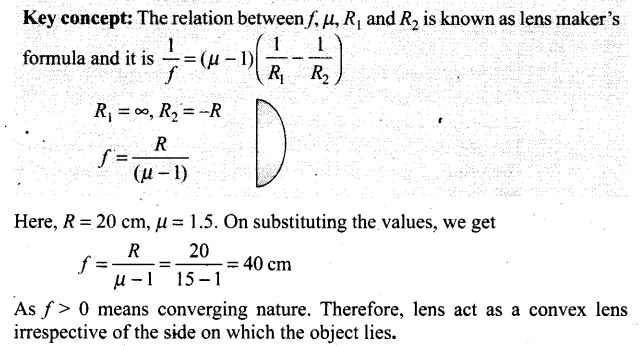Question 7. The phenomena involved in the reflection of radio waves by ionosphere is similar to
(a) reflection of light by a plane mirror
(b) total internal reflection of light in air during a mirage
(c) dispersion of light by water molecules during the formation of a rainbow
(d) scattering of light by the particles of air
Solution: (b) Radio waves are reflected by a layer of atmosphere called the Ionosphere, so they can reach distant parts of the Earth. The reflection of radio waves by ionosphere is due to total internal reflection. It is the same as total internal reflection of light in air during a mirage, i.e., angle of incidence is greater than critical angle.
Important point: The ionized part of the Earth’s atmosphere is known as the ionosphere. Ultraviolet light from the sun collides with atoms in this region knocking electrons loose. The creates ions, or atoms with missing electrons. This is what gives the Ionosphere its name- and it is the free electrons that cause the reflection and absorption of ratio waves.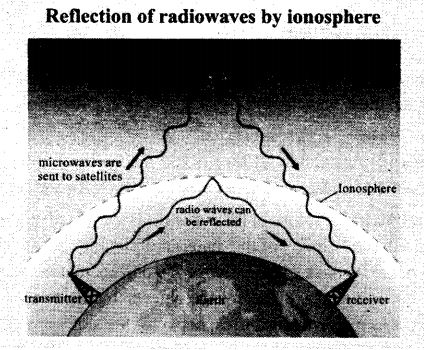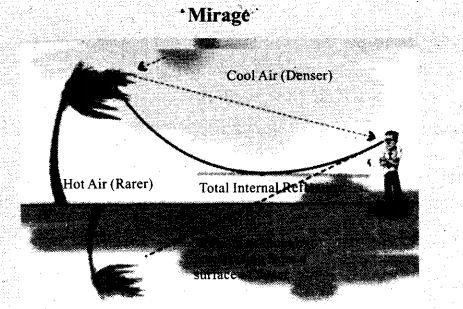Question 8. The direction of ray of light incident on a concave mirror is shown by PQ while directions in which the ray would travel after reflection is shown by four rays marked 1, 2, 3 and 4 (figure). Which of the four rays correctly shows the direction of reflected ray?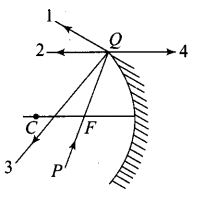(a) 1 (b) 2
(c) 3 (d) 4
Solution: (b) The ray PQ of light passes through focus F and incident on the concave mirror, after reflection, should become parallel to the principal axis and shown by ray 2 in the figure.
Important points:
We can locate the image of any extended object graphically by drawing any two of the following four special rays:
1. A ray initially parallel to the principal axis is reflected through the focus of the mirror (1).
2. A ray passing through the center of curvature is reflected back along itself (3).
3. A ray initially passing through the focus is reflected parallel to the principal axis (2).
4. A ray incident at the pole is reflected symmetrically.

Question 9. The optical density of turpentine is higher than that of water while its mass density is lower. Figure shows a layer of turpentine floating over water in a container. For which one of the four rays incident on turpentine in figure, the path shown is correct?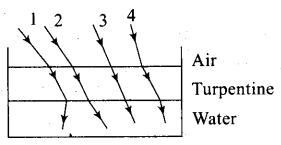(a) 1 (b) 2 (c) 3 (d) 4
Solution: (b)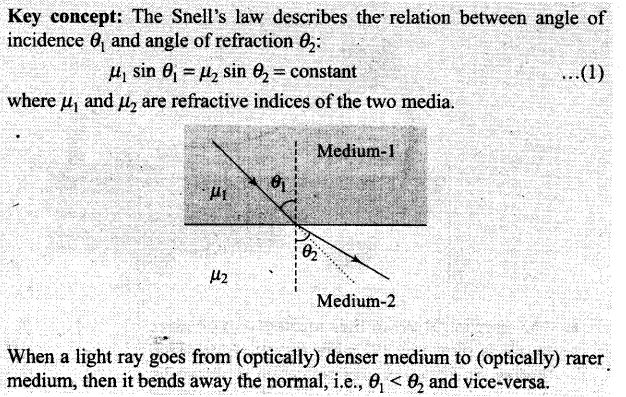Here, light ray goes from (optically) rarer medium air to optically denser medium turpentine, then it bends towards the normal, i.e., θ1 > θ2 whereas when it goes from to optically denser medium turpentine to rarer medium water, then it bends away the normal.

Question 10. A far is moving with a constant speed of 60 km h-1 on a straight road. Looking at the rear view mirror, the driver finds that the car following him is at a distance of 100 m and is approaching with a speed of 5 km h-1.
In order to keep track of the car in the rear, the driver begins to glance alternatively at the rear and side mirror of his car after every 2 s till the other car overtakes. If the two cars were maintaining their speeds, which of the following statement (s) is/are correct?
(a) The speed of the car in the rear is 65 km h-1
(b) In the side mirror, the car in the-rear would appear to approach with a speed of 5 km h-1 to the driver of the leading car
(c) In the rear view mirror, the speed of the approaching car would appear to decrease as the distance between the cars decreases
(d) In the side mirror, the speed of the approaching car would appear to increase as the distance between the cars decreases
Solution: (d)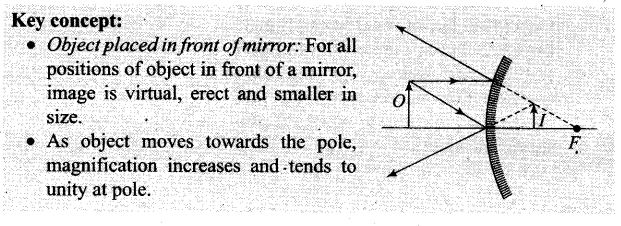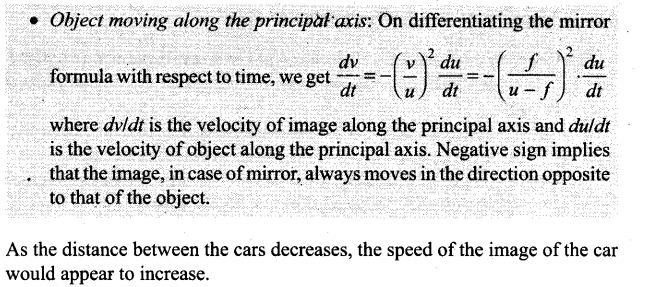Question 11. There are certain material developed in laboratories which have a negative refractive index figure. A ray incident from air (Medium 1) into such a medium (Medium 2) shall follow a path given by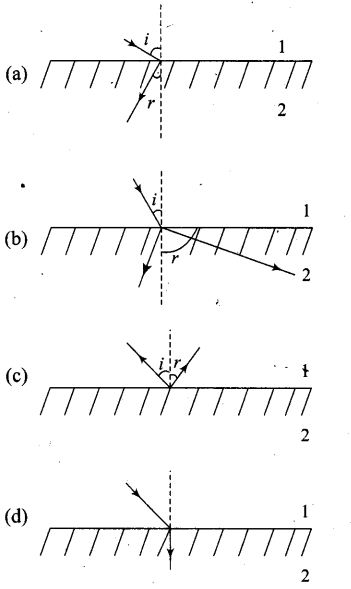Solution: (a) The materials with negative refractive index responds to Snell’s law just opposite way. If incident ray from air (Medium 1) incident on those material, the ray refract or bend same side of the normal as in option (a).

One or More Than One Correct Answer Type

Question 12. Consider an extended object immersed in water contained in a plane trough. When seen from close to the edge of the trough the object looks distorted because
(a) the apparent depth of the points close to the edge are nearer the surface of the water compared to the points away from the edge
(b) the angle subtended by the image of the object at the eye is smaller than the actual angle subtended by the object in air
(c) some of the points of the object far away from the edge may not be visible because of total internal reflection
(d) water in a trough acts as a lens and magnifies the object
Solution: (a, b, c)
Key concept: The light from the pencil is refracted when it passes from the water into air, bending away from the normal as it moves from high to low refractive index.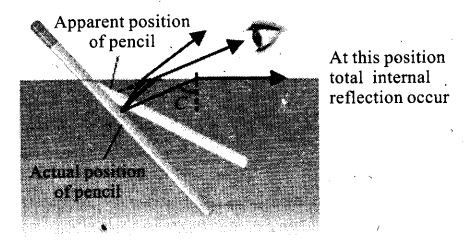When light from the submerged object before reaching to the observer gets, refracted from water surface, the rays bend away from normal and the angle subtended by the image of the object at the eye is smaller than the actual angle subtended by the object in air. Also the apparent depth of the .points close to the edge are nearer the surface of the water compared to the points away from the edge.
As we move towards right, the angle of incident increases and becomes equal to critical angle. Hence some of the points of the object far away from the edge may not be visible because of total internal reflection.

Question 13. A rectangular block of glass ABCD has a refractive index 1.6. A pin is placed midway on the face AB of figure. When observed from the face AD, the pin shall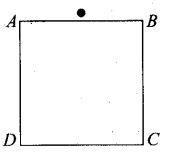(a) appear to be near A
(b) appear to be near D
(c) appear to be at the centre of AD
(d) not be seen at all
Solution: (a, d) As long as angle of incidence on AD of the ray emanating from pin is less than the critical angle, the pin shall appear to be near A.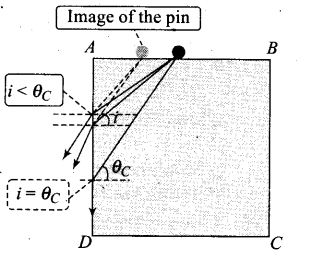When angle of incidence on AD of the ray emanating from pins is greater than the critical angle, the light suffers from total internal reflection and cannot be seen through AD.

Question 14. Between the primary and secondary rainbow, these is a dark band known as Alexandar’s dark band. This is because
(a) light scattered into this region interfere destructively
(b) there is no light scattered into this region
(c) light is absorbed in this region
(d)angle made at the eye by the scattered rays with respect to the incident light of the sun lies between approximately 42° and 50°
Solution: (a, d) The Alexandar’s dark band lies between the primary and secondary rainbows, formed due to light scattered into this region interfere destructively. The primary rainbows subtends an angle nearly 41° to 42° at observer’s eye, whereas secondary rainbows subtends an angle nearly 51° to 54° at observer’s eye w.r.t. incident light ray.
Hence, the scattered rays with respect to the incident light of the sun lies between approximately 42° and 50°.

Question 15. A magnifying glass is used, as the object to be viewed can be brought closer to the eye than the normal near point. This results in
(a) a larger angle to be subtended by the object at the eye and hence, viewed in greater detail
(b) the formation of a virtual erect image
(c) increase in the field of view
(d) infinite magnification at the near point
Solution: (a, b)
Key concept: A magnifying glass is a single convex lens of lesser focal length.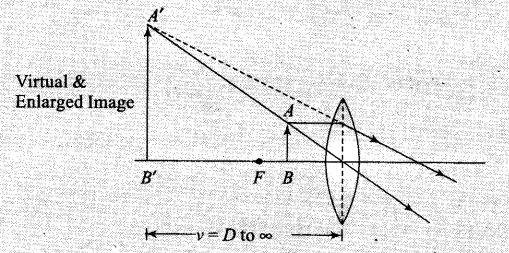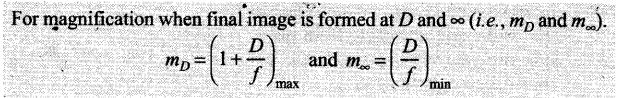When a magnifying glass is used, the object to be viewed can be brought closer to the eye than the normal near point. This results in a larger angle to be subtended by the object at the eye and hence, viewed in greater detail. Moreover, the formation of a virtual erect and enlarged image takes place.

Question 16. An astronomical refractive telescope has an objective of focal length 20 m and an eyepiece of focal length 2 cm.
(a) The length of the telescope tube is 20.02 m
(b) The magnification is 1000
(c) The image formed is inverted
(d) An objective of a larger aperture will increase the brightness and reduce chromatic aberration of the image
Solution: (a, b, c)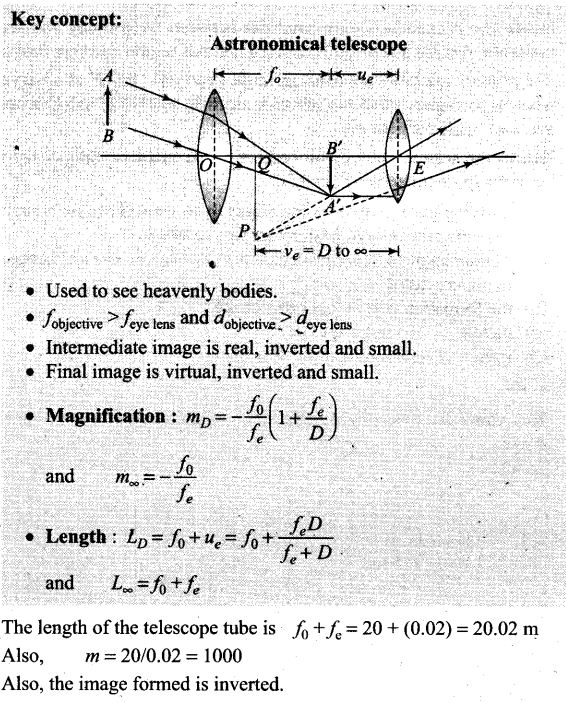Question 17. Will the focal length of a lens for red light be more, same or less than that for blue light?
Solution: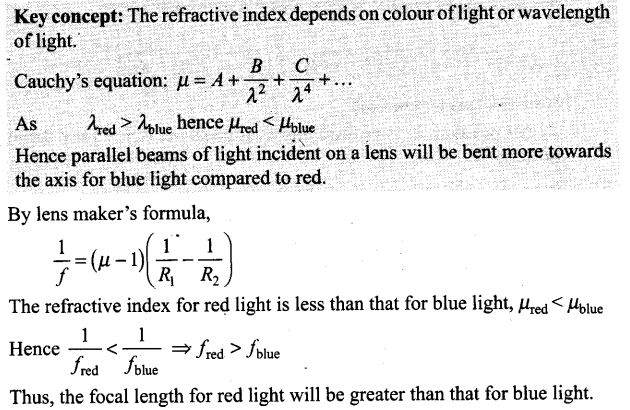Question 18. The near vision of an average person is 25 cm. To view an object with an angular magnification of 10, what should be the power of the microscope?
Solution: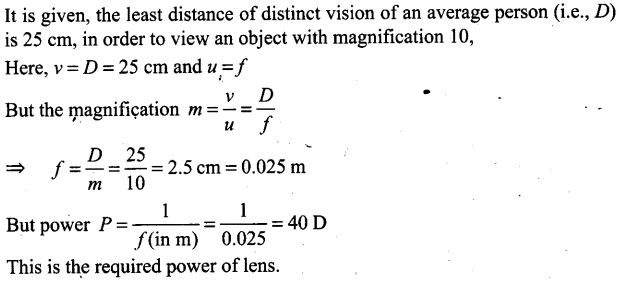Question 19. An unsymmetrical double convex thin lens forms the image of a point object on its axis. Will the position of the image change if the lens is reversed?
Solution: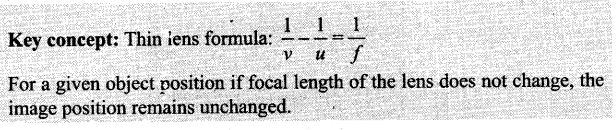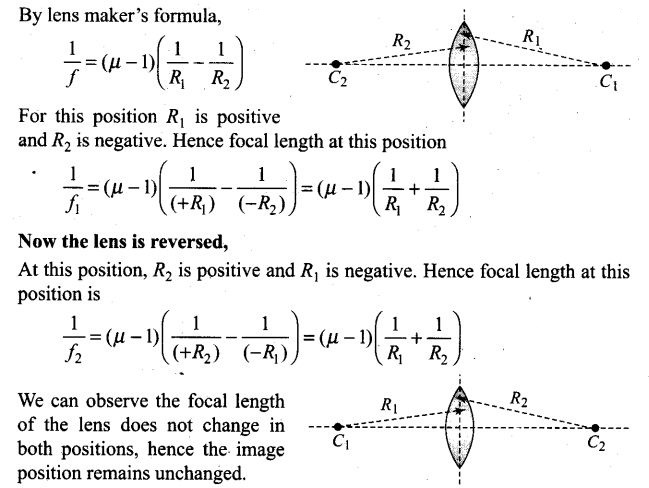Question 20. Three immiscible liquids of densities d1> d2> d3 and refractive indices μ1> μ2> μ3 are put in a beaker. The height of each liquid column is h/3. A dot is made at the bottom of the beaker. For near normal vision, find the apparent depth of the dot.
Solution: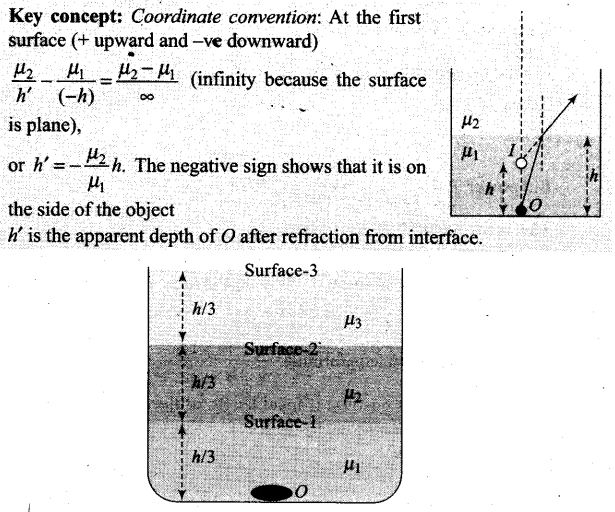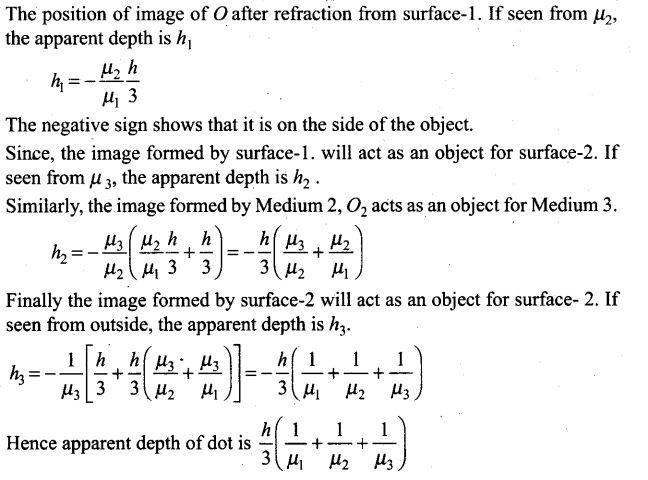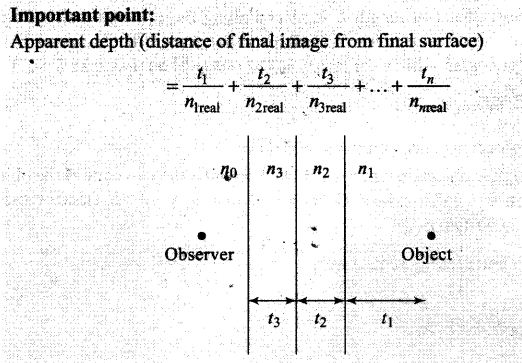Question 21. For a glass prism (μ= √3 ), the angle of minimum deviation is equal to the angle of the prism. Find the angle of the prism.
Solution: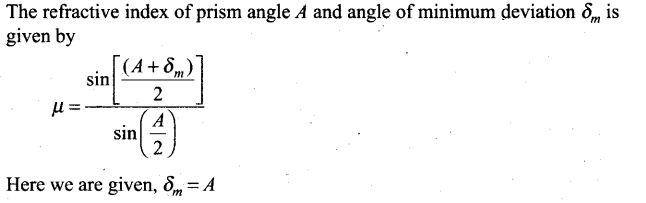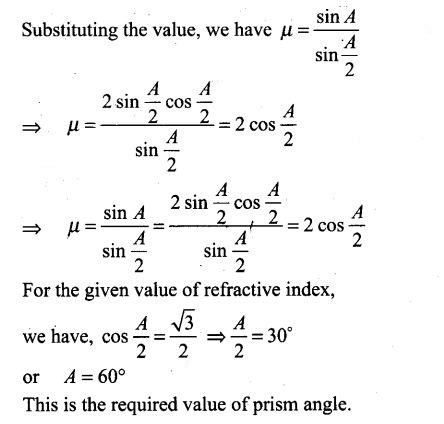Question 22. A short object of length L is placed along the principal axis of a concave mirror away from focus. The object distance is u. If the mirror has a focal length f what will be the length of the image? You may take L << |v – f |.
Solution: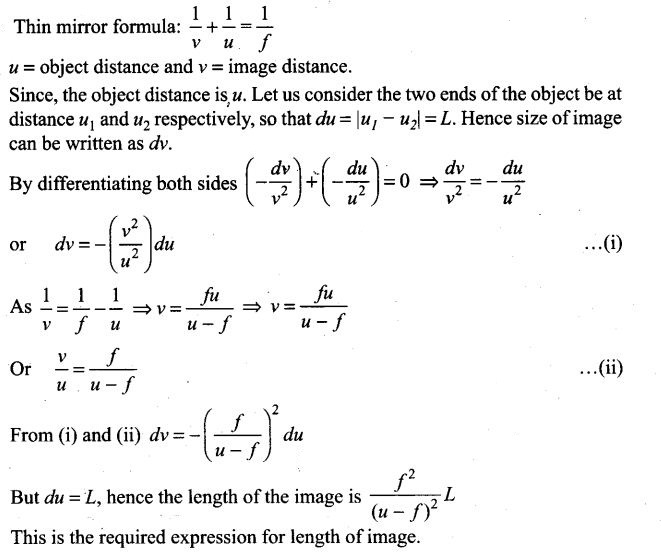Question 23. A circular disc of radius R is placed co-axially and horizontally inside an opaque hemispherical bowl of radius a (figure). The far edge of the disc is just visible when viewed from the edge of the bowl. The bowl is filled with transparent liquid of refractive index μ. and the near edge of the disc becomes just visible. How far below the top of the bowl is the disc placed?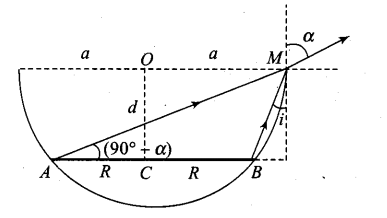Solution: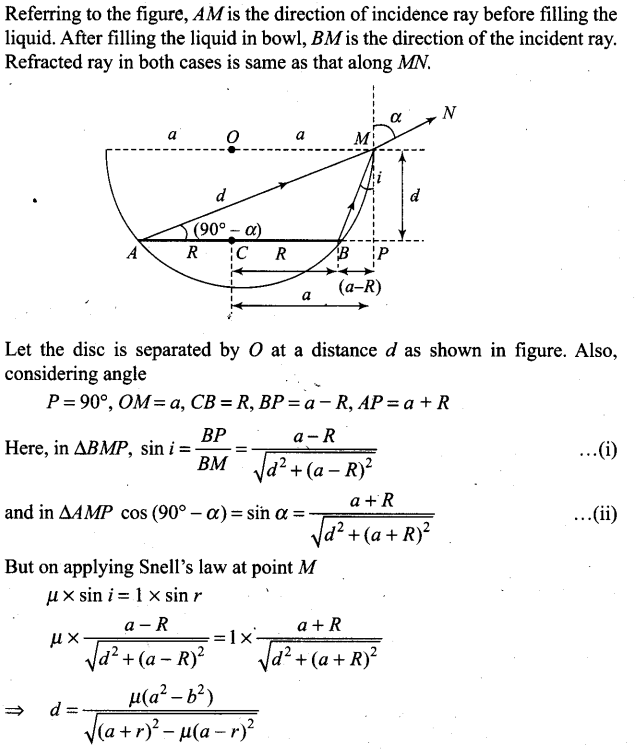Question 24. thin convex lens of focal length 25 cm is cut into two pieces 0.5 cm above the principal axis. The top part is placed at (0, 0) and an object is placed at (-50 cm, 0). Find the coordinates of the image.
Solution: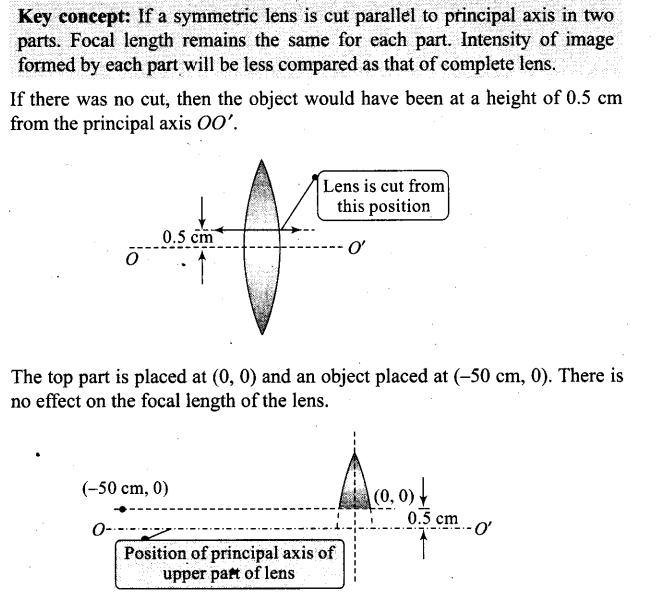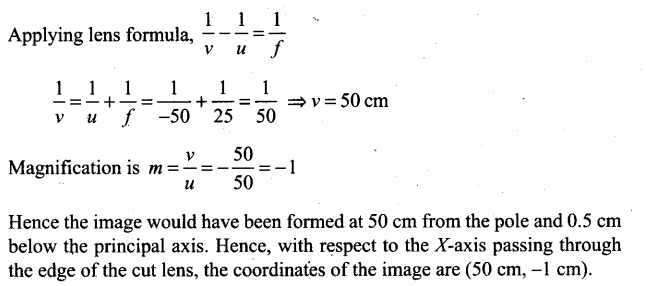Question 25. In many experimental set-ups, the source and screen are fixed at a distance say D and the lens is movable. Show that there are two positions for the lens for which an image is formed on the screen. Find the distance between these points and the ratio of the image sizes for these two points.
Solution:
Key Concept: This is also one of the methods for finding focal length of the length in laboratory and knows as “Displacement method”.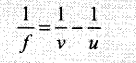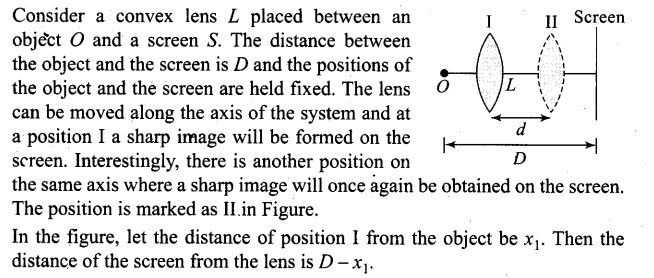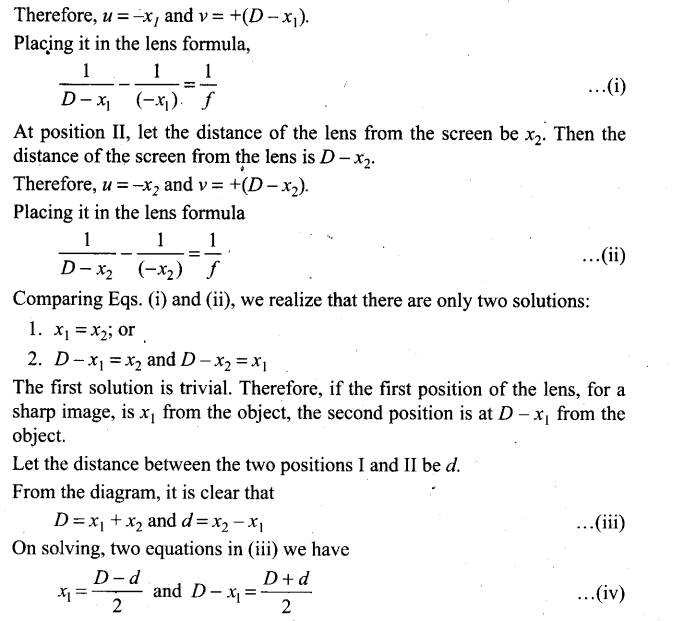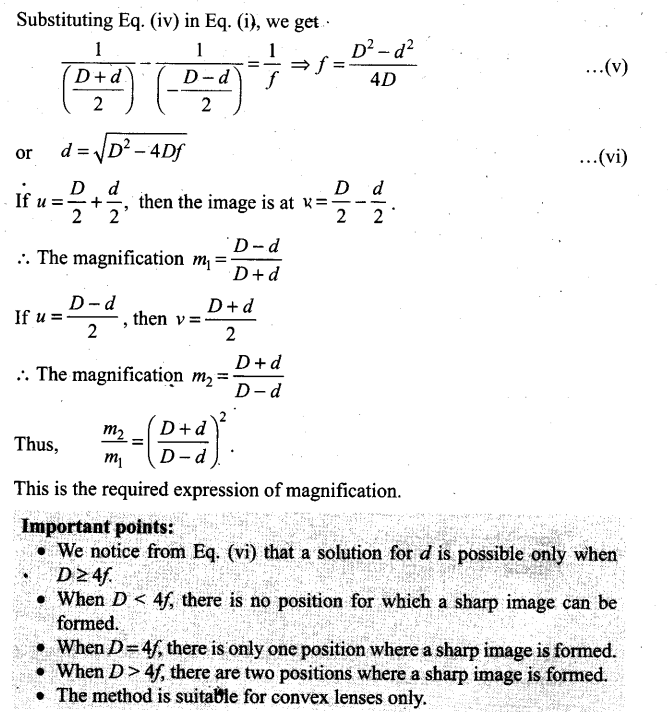Question 26. A jar of height h is filled with a transparent liquid of refraction index μ (figure).At the center of the jar on the bottom surface is a dot.Find the minimum diameter of a disc,such that when placed on the top surface symmetrically about the center,the dot is invisible.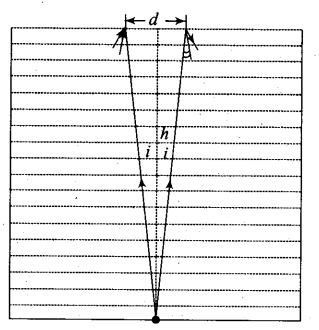Solution: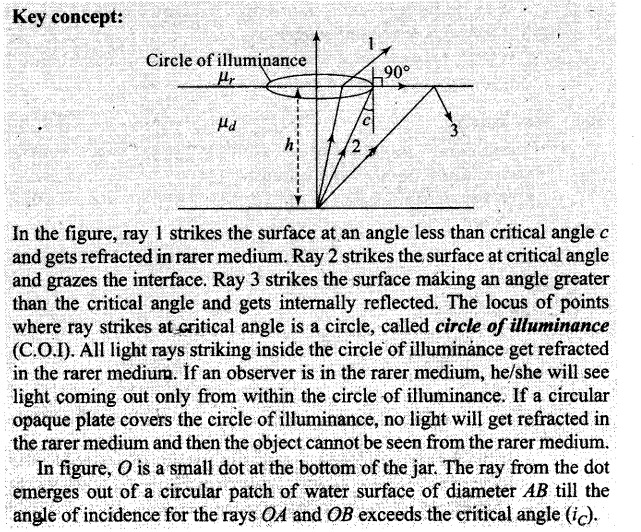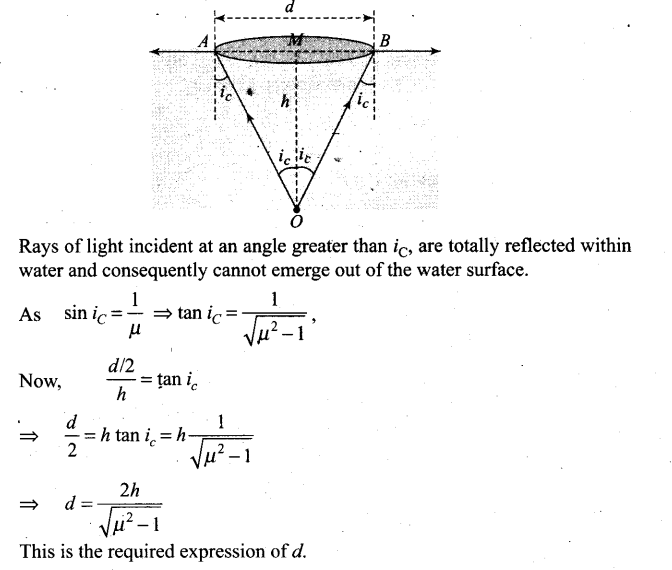Question 27. A myopic adult has a far point at 0.1 m. His power of accommodation is 4 D.
(i) What power lenses are required to see distant objects?
(ii) What is his near point without glasses?
(iii) What is his near point with glasses? (Take the image distance from the lens of the eye to the retina to be 2 cm.)

Solution: Key concepts: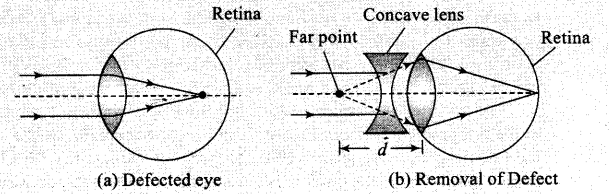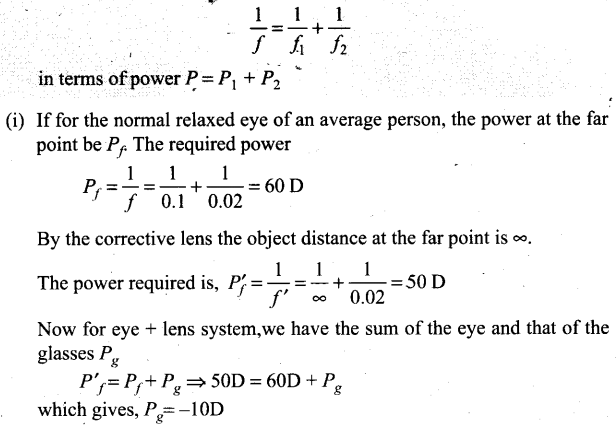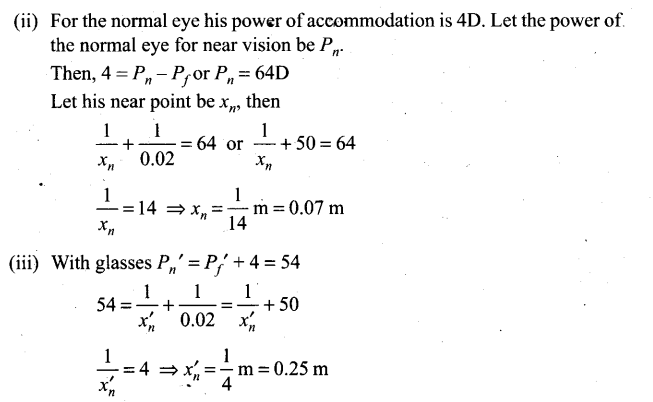Question 28. Show that for a material with refractive index μ ≥ √2, light incident at angle shall be guided along, a length perpendicular to the incident face.
Solution: Let the ray incident on face AB at angle i, after refraction, it travels along PQ and then interact with face AC which is perpendicular to the incident face.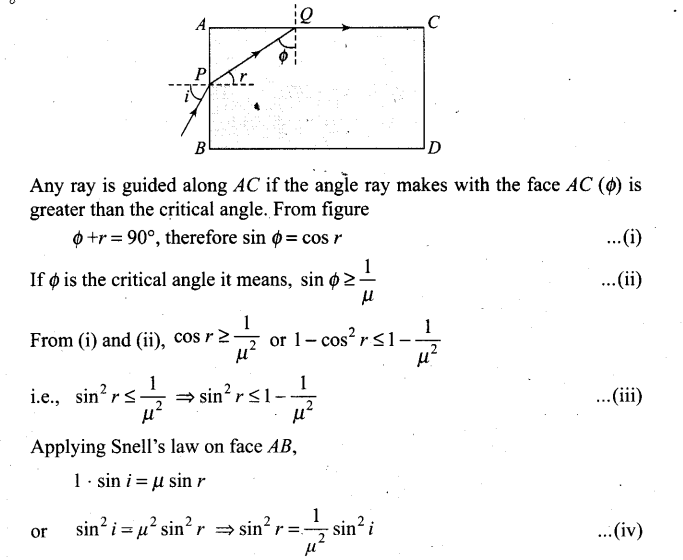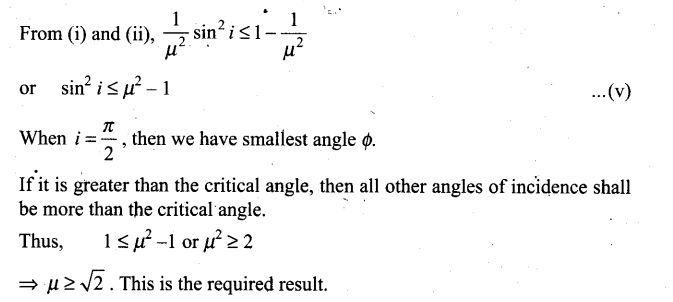Question 29. The mixture of a pure liquid and a solution in a long vertical column (i.e., horizontal dimensions << vertical dimensions) produces diffusion of solute particles and hence a refractive index gradient along the vertical dimension. A ray of light entering the column at right angles to the vertical is deviated from its original path. Find the deviation in travelling a horizontal distance d << h, the height of the column.
Solution: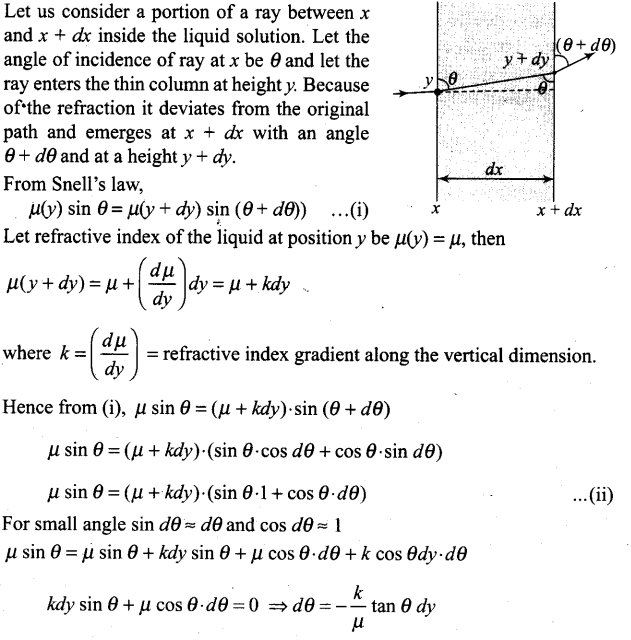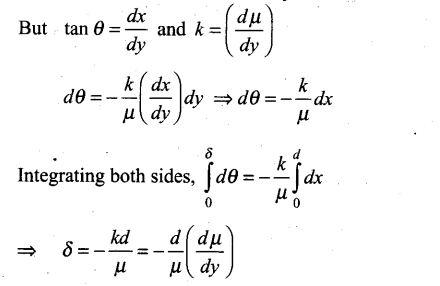Question 30.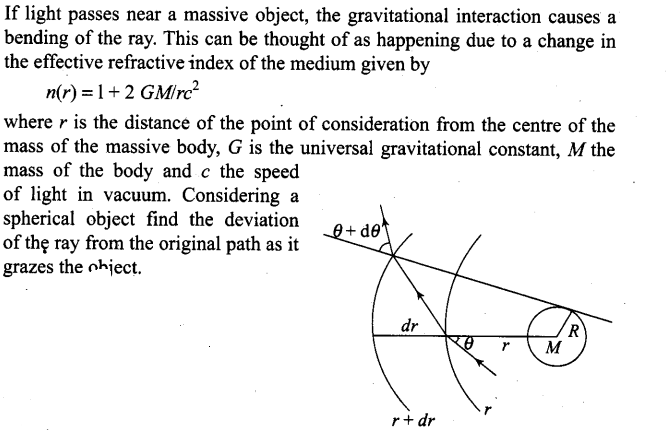Solution: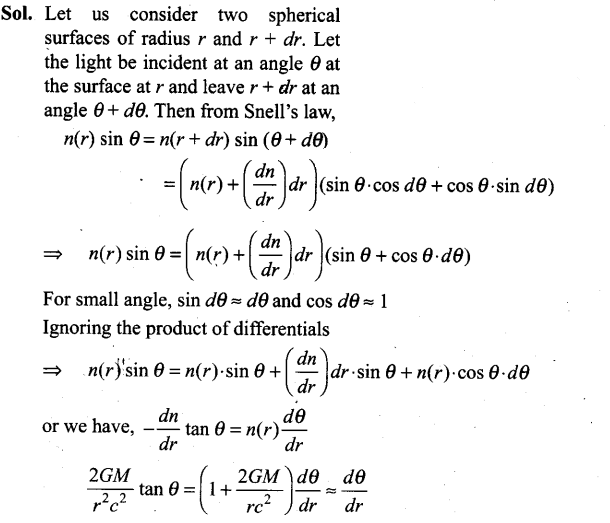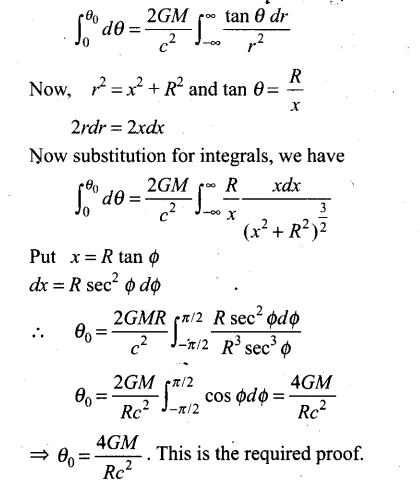Question 31. An infinitely long cylinder of radius R is made of an unusual exotic material with refractive index -1 (figure). The cylinder is placed between two planes whose normals are along the y-direction. The centre of the cylinder O lies along they-axis.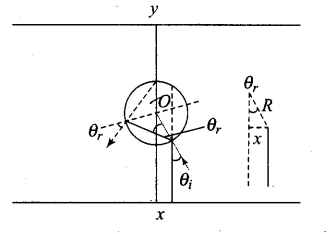A narrow laser beam is directed along the y-direction from the lower plate.
The laser source is at a horizontal
distance x from the diameter in the y-direction. Find the range of x such that light emitted from the lower plane does not reach the upper plane.
Solution: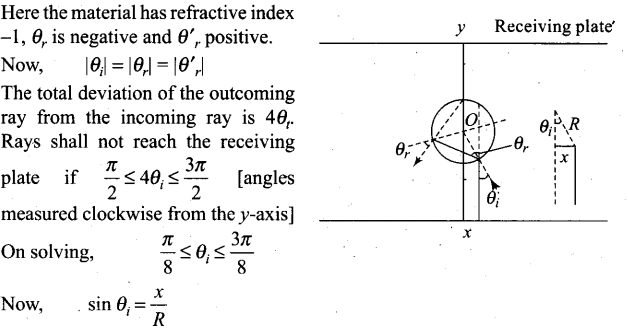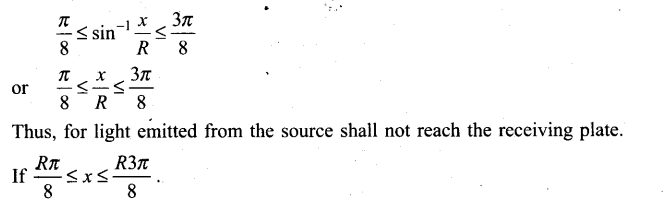Question 32. (i) Consider a thin lens placed between a source (S) and an observer (O)
(Figure). Let the thickness of the lens vary as w(b) =w0– b2/α , where b is the vertical distance from the pole, w0 is a constant. Using Fermat’s principle, i.e., the time of transit fora ray between the source and observer is an extremum find the condition that all paraxial rays starting from the source will converge at a point O on the axis. Find the focal length.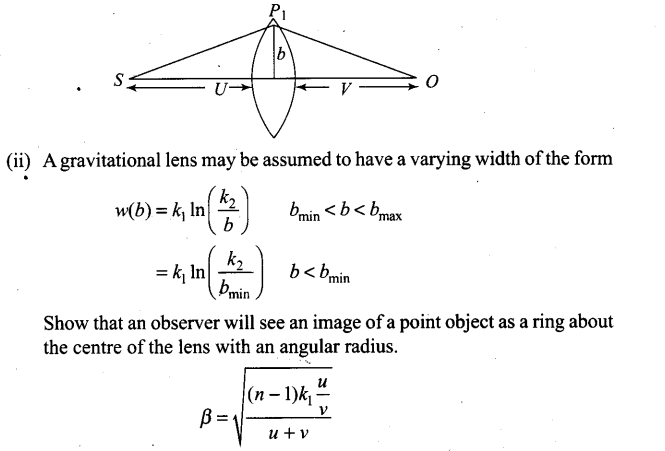Solution: